Today we can see the usage of Artificial Intelligence (AI) in almost every industry. This includes working with data that comes from a different source, thus it has a different format and different nature.

With the huge amount of information created every day we can see an exponential increase in the usage of many Big Data technologies that can help us manipulate with this data.

This increased usage of Big Data technologies, bring the popularity of manipulating with the data in real-time. This way most of the results we get after doing different operations over the data, are time-dependent.

With the information being time-dependent we have the ability to predict what value can that information has in some date point in the future, based on the value that the information had in the past, and the value that it has now. This ability has usage in many different scenarios, like predicting the usage of electricity, predicting the traffic of a web site, predicting the numbers of sales of a certain product, predicting stock prices, etc.

These cases of predicting the value of information in some time point are part of one field of the AI, called Time series. We are going to make few posts about this field in the future, since most of our projects so far are hugely influenced by working with time-dependent data.

Our mission on this post is to help you with preparing the time-date data on which the value of the information depends, so you can organize it in a format that will result in better solutions to the problem you are trying to solve.

## Working with date-time data

import datetime
import numpy as np
import pandas as pd
from dateutil.parser import parse
import pytz

time_stamps = [‘2015-03-08 10:30:00.360000+00:00’, ‘2017-07-13 15:45:05.755000-07:00’,

‘2012-01-20 22:30:00.254000+05:30’, ‘2016-12-25 00:30:00.000000+10:00’]

df = pd.DataFrame(time_stamps, columns=[‘Time’])
print(df)

To work with date-time data, we need a few libraries. Obviously we are going to use the DateTime library which will help us do different conversions to date-time format, then we are going to use the NumPy library which is adding support for large, multi-dimensional arrays and matrices, along with a large collection of high-level mathematical functions to operate on these arrays, then pandas which offers data structures and operations for manipulating numerical tables and time series, dateutil which can parse time data and pytz which allows accurate and cross platform timezone calculations.

From the code above, we are giving a list of string in a date-time format understandable for Python. Using the pandas library we are making the frame of this data, which will create a matrix-like structure of four rows and one column named “Time”.

### The output of this code is: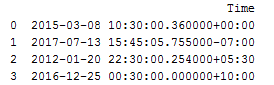ts_objs = np.array([pd.Timestamp(item) for item in np.array(df.Time)])
df[
‘TS_obj’] = ts_objs
print(“Timestamp converts:\n)
print(ts_objs)
print(“Data frame:\n)
print(df)

With this code, we are converting the data in the timestamp type. We are creating a matrix of the item in the frame, and then creating a new column in the data frame named “TS_obj” where we are going to add the timestamp data.

### The output of the code above is: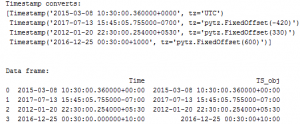From the output, we can see that timestamp data has few components like the date, the time and the time zone. For the purpose of keeping things simple, we are going to show you how can you work with date based features, and with time-based features, since most of the projects we’ve worked on are dependent on one or both of these formats.

## Working with date data

Each timestamp type value has a date component that can be used to extract useful information and features pertaining to the date. These include features and components like year, month, day, quarter of the year, day of the week, day name, etc.

df[‘Year’] = df[‘TS_obj’].apply(lambda x: x.year)
df[
‘Month’] = df[‘TS_obj’].apply(lambda x: x.month)
df[
‘Day’] = df[‘TS_obj’].apply(lambda x: x.day)
df[
‘Quarter’] = df[‘TS_obj’].apply(lambda x: x.quarter)
df[
‘DayOfWeek’] = df[‘TS_obj’].apply(lambda x: x.dayofweek)
df[
‘DayName’] = df[‘TS_obj’].apply(lambda x: x.weekday_name)
df[[
‘Time’, ‘Year’, ‘Month’, ‘Day’, ‘Quarter’, ‘DayOfWeek’, ‘DayName’]]
pd.set_option(
‘display.max_rows’, 500)
pd.set_option(
‘display.max_columns’, 500)
pd.set_option(
‘display.width’, 1000)
print(“Data frame:”)
print(df)

With the code above we are taking the features we mentioned and we are getting the following output: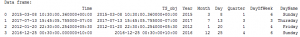## Working with time data

Each timestamp type value also has a time component that can be used to extract useful information and features pertaining to the time. These include attributes like hour, minute, second, microsecond, etc.

df[‘Hour’] = df[‘TS_obj’].apply(lambda d: d.hour)
df[
‘Minute’] = df[‘TS_obj’].apply(lambda d: d.minute)
df[
‘Second’] = df[‘TS_obj’].apply(lambda d: d.second)
df[
‘MUsecond’] = df[‘TS_obj’].apply(lambda d: d.microsecond)
df[[
‘Time’, ‘Hour’, ‘Minute’, ‘Second’, ‘MUsecond’]]
print(“Data frame:”)
print(df)

With the code above we are taking the features we mentioned and we are getting the following output: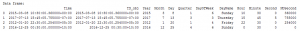## Example of advanced feature extraction

In the two previous parts, we had some basic features extraction on timestamp data. Obviously we can do some further feature extraction on already extracted features.

For this example, we are going to do use binning to bin each temporal value into a specific time of the day by leveraging the Hour feature we obtained in the previous part.

hour_bins = [1, 5, 11, 16, 21, 23]
bin_names = [
‘Late Night’, ‘Morning’, ‘Afternoon’, ‘Evening’, ‘Night’]
df[
‘TimeOfDayBin’] = pd.cut(df[‘Hour’],
bins=hour_bins, labels=bin_names)
df[[
‘Time’, ‘Hour’, ‘TimeOfDayBin’]]
print(“Data frame”)
print(df)

The following output is obtained by creating a list of integer values named “hour_bins”, these bins are representing a ranges where the values of the “Hour” feature needs to belong in order to be labeled with one of the labels in the “bin_names” array. Then we are creating a new column named “TimeOfDayBin”, and by using the “cut” function of the pandas library we are doing the labeling.

## This is the output we get: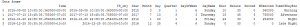## Conclusion

This post is a small example of how can you organize a date-time data in order to get wanted solution on a certain problem. When you are trying to build a Machine Learning model, the most important part is finding proper data and preparing it in order to feed it to your model.

In some of the next posts, we are going to explain to you how can you do feature extraction on text data if you are working with Natural Language Processing and feature extraction on image data if you are working with Image Processing.

We hope that we spark a little interest in you so you will learn about feature extraction on data since this technique is a vital skill of every successful Data Scientist.

Like with every post we do, we encourage you to continue learning, trying and creating.